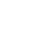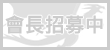LV. 34
GP 357# 只要我長大 (nadia46, 霜玉貓兒)

[b]只要我長大[/b] (情人節徵文)

=^.^=~=^.^=~=^.^=~=^.^=~=^.^=~=^.^=~=^.^=~=^.^=~=^.^=~=^.^=~=^.^=~=^.^=

「嘿嘿……讓你瞧瞧我的厲害！」具有紫髮紫瞳的小男孩踢出一顆球，而這顆球不偏不倚地往拉杜頭上砸去。

「喔！好痛！是誰踢這顆球的？」拉杜左手揉了揉被球打到的後腦勺，右手拿著球問。

「是我，大哥哥。」小男孩好整以暇地從樹叢中走出。

「你……你……你……你是誰？」眼見前一刻還氣得滿臉通紅的拉杜，後一刻表情卻突然變得一臉疑惑地問著，小男孩當場傻眼。

「嗯……我平常應該是沒做什麼壞事啊？況且現在是大白天，月光應該很弱吧……咦？小弟弟跑哪去了？」趁拉杜還在思考小男孩話中之意時，小男孩早就腳底抹油、拔腿就跑啦！

「可惡！我被騙了！」原地，只剩下氣得跺腳的拉杜。

=^.^=~=^.^=~=^.^=~=^.^=~=^.^=~=^.^=~=^.^=~=^.^=~=^.^=~=^.^=~=^.^=~=^.^=

「哇哈哈……天底下怎麼會有這麼笨的人！呼……哈哈……」男孩邊喘氣，邊持續笑著。

「拉亞，瞧你笑成這樣，你是不是又去做什麼壞事了？」說這話的，是寶路花三姊妹中的小妹─芝華。由於大姐溫迪去醫院看病，二姊瑪露在學校參加社團活動，所以此刻是由芝華在看店。

「你應該學學你妹妹瑪亞，你看她多乖呀！」芝華衝去坐在店內吃藍莓慕斯的瑪亞身邊，戳戳她的臉。

「唉唷！芝華姊姊，妳別這樣……」跟拉亞一樣具有紫髮紫瞳、綁著雙馬尾的小女孩瑪亞，正是拉亞的妹妹。由於雙方父母是世交，所以拉亞瑪亞兩兄妹跟寶路花三姊妹的感情甚好，平時兩人常常會來光顧田園茶館。

「哼！芝華姊姊偏心！」拉亞看見芝華對他妹妹那麼好，雙手在胸前交岔，以示不滿。

「好啦好啦，拉亞乖，趕快去洗手，洗完手來吃點心吧！」可惜的是芝華完全不知情，她摸摸拉亞的頭，只把他當小弟弟看待。

「哥哥，你剛剛是在笑什麼啊？」雖知道八九不離十是跟整傳言中芝華喜歡的對象─拉杜有關，但是瑪亞想知道她哥哥是怎麼整對方的。

「妳想知道？耳朵靠過來。」拉亞鬼鬼祟祟地在瑪亞耳邊說悄悄話。

「噗～結果他真相信啊？」瑪亞在一旁咯咯笑個不停。

「是啊是啊，很蠢吧！」拉亞想起剛剛拉杜一臉拙樣，就止不住笑意。

「光這樣還不夠呢！妹妹，我接下來要交代妳一件神聖的任務！」拉亞的眼神中充滿著算計的意味，緩緩地從口袋中拿出一支簽字筆。

「不會吧……哥哥，那落伍了啦！」瑪亞不愧是拉亞的妹妹，拉亞話都還沒說，她就已經知道他想做什麼。

「你們這兩個小鬼頭從剛剛就在偷偷摸摸說些什麼啊？」芝華雙手插腰地加入兩人的話題，十足有媽媽的架勢。

「呃……沒什麼、沒什麼……」兩人很有默契地結束話題。

「糟！快跑！」拉亞本想從窗邊逃走的，但眼明手快的芝華馬上就抓住了他。

「親愛的拉亞小弟弟，我想你還欠我一個解釋吧……說，這是怎麼一回事呀？」說這話時，芝華向拉亞投以一個好甜好甜的笑容。

（可惡的妹妹……關鍵時刻都幫不上忙……）拉亞心想。

「原來是這樣……拉亞呀……整拉杜這種事只有我可以做，知道嗎？」芝華繼續甜甜地笑著，不過對象換成了拉杜。

「啥！？」拉杜此時看見芝華的笑臉，卻沒來由地打起冷顫來。

「為什麼就只有妳能欺負他，那是因為妳喜歡他！所以妳想獨占他對不對？」拉亞受夠了，他不想芝華老把他當小孩子看待！

「哥哥！」一旁的瑪亞也急了，她沒想到今天的拉亞特別的……呃……大膽！？

「呃……拉亞……你知道你剛剛說了什麼嗎？」芝華懷疑剛剛那句話真的是出自一個七、八歲小男孩的嘴巴嗎？

「我知道，也很清楚自己說了些什麼。因為妳認為我只是小孩子，所以根本不了解我的看法，也不了解其實我很喜歡妳的！」此刻的拉亞，就如同脫韁的野馬一般，激動地說著。

「啊！？喔……我也很喜歡你啊！」芝華原先愣了一下，後來她認為那只是因為拉亞看太多連續劇，受其影響而有樣學樣，所以她開玩笑式地回應著拉亞。

「妳只是在開玩笑的！妳根本就不是真心的！！！」拉亞看到芝華對於他的心意完全不當一回事，憤怒地離開。

「哥哥……等等啊！」瑪亞想要去追拉亞，卻被芝華阻止了。

「讓他去吧……過一陣子他的心情平復，自然就會回來了。」芝華依舊認為拉亞是在耍任性。

「芝華姊姊，哥哥他……是真的喜歡妳呀！」瑪亞認真地對芝華說著，之後往門外衝去。

「芝華，我想……我該走了……」從頭到尾像是局外人的拉杜，這時也好不容易找回自己的聲音，跟芝華道別。

=^.^=~=^.^=~=^.^=~=^.^=~=^.^=~=^.^=~=^.^=~=^.^=~=^.^=~=^.^=~=^.^=~=^.^=

「妹妹，回魂啊！」瑪露兩手抓著芝華的肩膀搖著。

「啊……這不是肯德基！！！」回魂後的芝華突然大喊，嚇了瑪露一跳。

「妹妹……妳還好吧？是不是生病了？要不要去看醫生？」瑪露趕緊將手往芝華額頭上摸去，看看她有沒有發燒。

「沒……沒事啦！呵呵……」芝華試圖以乾笑掩飾自己剛剛的失態，但這招對瑪露是沒用的。

「是嗎？妹妹，妳要是有心事的話，我想還是說出來比較好，說不定我可以提供妳一些建議喔！」瑪露對芝華投以會心一笑，想使芝華安心一些。

「喔……原來如此啊！」瑪露聽完後笑了笑，了然似的點了點頭。

「姊，妳認為那麼小的小孩子知道什麼是情愛嗎？」正所謂「事不關己則已，關己則亂」。平時看來精明的芝華，此時卻茫然不知所措。

「我認為拉亞是知道的，今非昔比，現在的小孩都很早熟的。我想，更重要的應該是妳對拉亞的感覺吧！妳喜歡拉亞嗎？」瑪露專注地看著芝華，認真地問。

「我……我不知道……」因為事出突然，芝華的腦袋仍舊呈現一片漿糊狀態。

「妳好好想想吧……對了！再過幾天就是情人節了，我得去準備巧克力了。」瑪露對芝華嫣然一笑後，轉身走向廚房。

=^.^=~=^.^=~=^.^=~=^.^=~=^.^=~=^.^=~=^.^=~=^.^=~=^.^=~=^.^=~=^.^=~=^.^=

「情人節給我去死去死！！！」

「那是誰家的小孩呀！真沒教養……」

「好可憐喔！還這麼小，精神就不正常了……」等諸如此類的話。

「妹妹，妳為何跑得這麼喘？」看見瑪亞匆匆忙忙地跑來，拉亞不解地問。

「田園茶館爆炸，芝華……芝華姊姊她……受傷而被送去醫院……」瑪亞邊喘著氣邊說著。

「甚麼！？妹妹妳快帶我去醫院！」聽到自己的心上人受傷送醫，縱使前一刻還在生她的氣，這時也早已忘了一乾二淨，心急如焚地跟瑪亞趕往醫院。

=^.^=~=^.^=~=^.^=~=^.^=~=^.^=~=^.^=~=^.^=~=^.^=~=^.^=~=^.^=~=^.^=~=^.^=

「雖然這次田園茶館爆炸好像是一場意外，不過為了以防萬一，我還是問一下，芝華，妳在廚房時有沒有發現什麼異狀？或是妳推測有可能造成這次爆炸的原因為何？」身為費拿魯城警局局長的雅史，不論何時都保持著理性的態度處理事情。

「嗯……是沒發現什麼異狀啊……啊！這次我做巧克力時，跟貓兒拿了這個……」芝華邊說，邊將一包物品拿出，上面寫著「獨門戀愛秘方─核融可可亞粉」。

「貓兒！？」其他人異口同聲的大叫著，再看了一下芝華拿出的物品後，眾人瞬間明瞭這次爆炸事件的「肇事者」是誰。

「哥哥，我想先去一下廁所。」瑪亞在他們走後，也很識趣地離開。

「對不起！你的巧克力我來不及做好送給你！」

「對不起！我不應該耍脾氣的！」

「我果然只是個小孩子，不夠成熟……現在的我，還配不上妳。妳可以等我長大嗎？我長大後一定會成為一個溫柔體貼的好男人，並且賺很多很多的錢，讓妳能過著幸福且不愁吃穿的日子！」兩人笑完後，拉亞對芝華認真地說著，眼神中帶有百分之百的誠懇。

「可以！不過要我等你長大的話，我有個條件！」芝華偏著頭，眼珠子轉了轉後對拉亞說著。

「什麼條件？」雖然這麼問著，不過拉亞心想不管芝華開什麼條件他都會答應的。

「就是……在你長大之前，只有我能整你！當然，你長大後也一樣！」芝華促狹地對拉亞眨了一下眼。

「沒問題！」拉亞的笑容中，似乎帶有小惡魔的氣息。

=^.^=~The End~=^.^=

=^.^=~=^.^=~=^.^=~=^.^=~=^.^=~=^.^=~=^.^=~=^.^=~=^.^=~=^.^=~=^.^=~=^.^=

(天音：「應該說是妳寫的言情小說都很奇怪吧……」)

[s]也請同為「去死去死軍團」的團員繼續爲情人節去死去死努力!XD(毆)[/s]face基於日前微軟官方表示 Internet Explorer 不再支援新的網路標準，可能無法使用新的應用程式來呈現網站內容，在瀏覽器支援度及網站安全性的雙重考量下，為了讓巴友們有更好的使用體驗，巴哈姆特即將於 2019年9月2日 停止支援 Internet Explorer 瀏覽器的頁面呈現和功能。

。Google Chrome（推薦）
。Mozilla Firefox
。Microsoft Edge（Windows10以上的作業系統版本才可使用）

face我們了解您不想看到廣告的心情⋯ 若您願意支持巴哈姆特永續經營，請將 gamer.com.tw 加入廣告阻擋工具的白名單中，謝謝 !【教學】